# Intermediate Geometry : How to find the equation of a line

## Example Questions

1 2 4 Next →

### Example Question #31 : How To Find The Equation Of A Line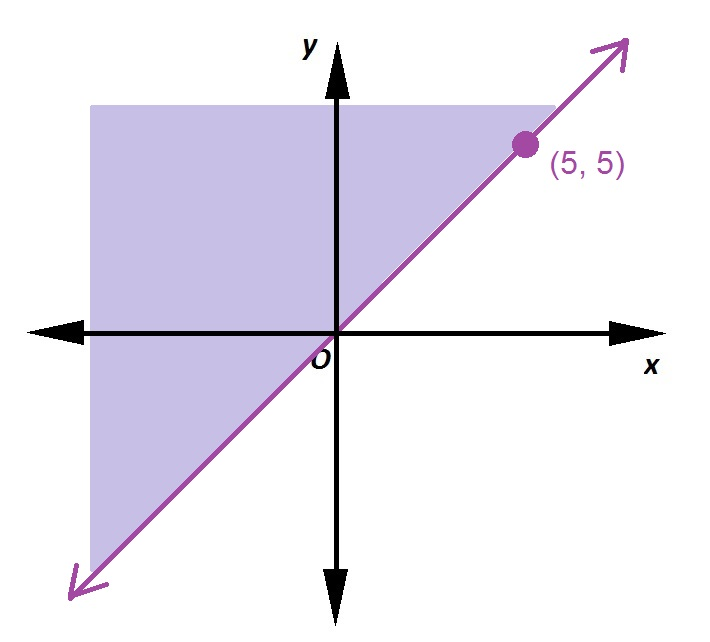Which inequality is graphed above?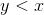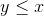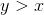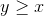Explanation:

The line that serves as the boundary passes through the points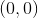and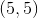and can easily be seen to be the line of the equation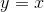.

The graph of the inequality includes the line itself, as is demonstrated by the fact that it is solid, so the correct choice is eitherorFrom the diagram, we see that, for example, the point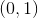(and the rest of the positive-axis) is in the set. Since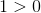, this makes the statementtrue, so that is the inequality to choose.

### Example Question #32 : How To Find The Equation Of A Line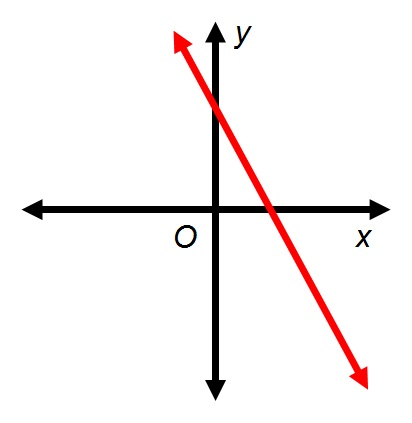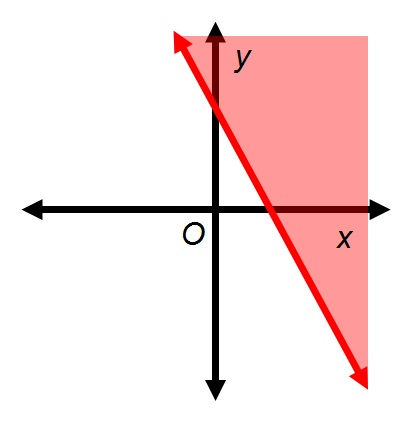Refer to the above figures. To the left is the graph of the equation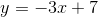What inequality is graphed at right?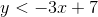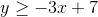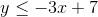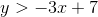Explanation:

As indicated by the solid line, the graph of the inequality at right includes the line of the equation, so the inequality graphed is eitherorTo determine which one, we can select a test point and substitute its coordinates in either inequality, testing whether it is true for those values. The easiest test point is; it is not part of the solution region, so we want the inequality that it makes false. Let us select the first inequality: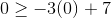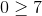makes this inequality false, so the graph of the inequalityis the one that does not include the origin. This is the correct choice. (Note that if we had selected the other inequality, we would have seen thatmakes it true; this would have allowed us to draw the same conclusion.)

### Example Question #33 : How To Find The Equation Of A Line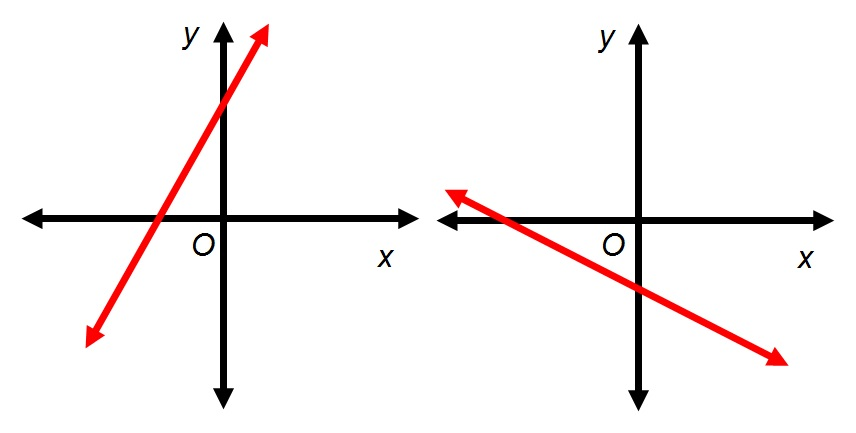Refer to the above diagrams. At left is the graph of the equation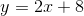At right is the graph of the equation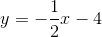Which of the following is a graph of the system of linear inequalities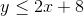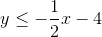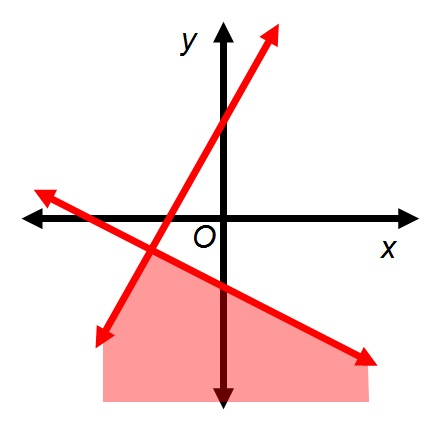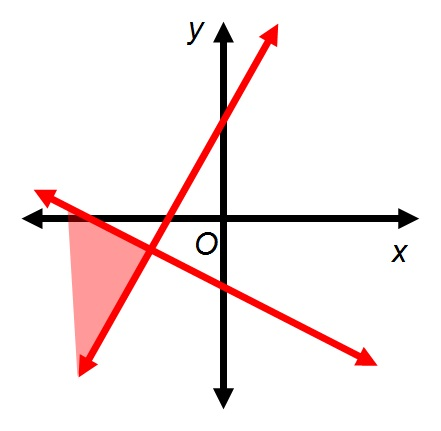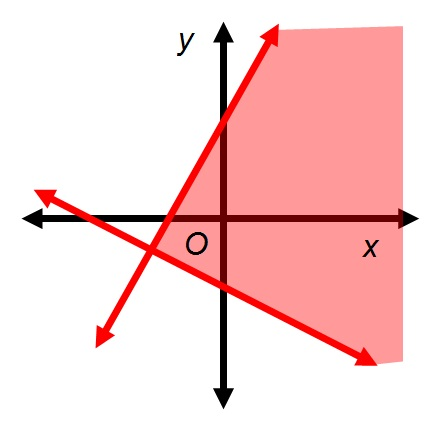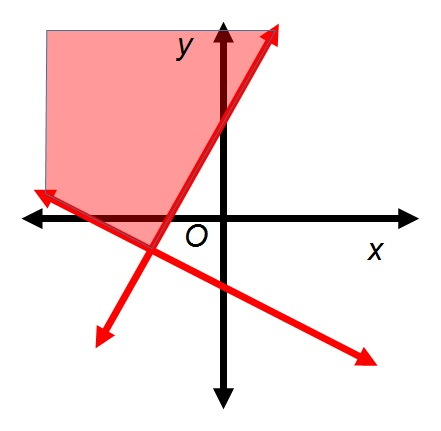Explanation:

The graph of a linear inequality that includes either the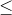or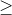symbol is the line of the corresponding equation along with all of the points on either side of the line. We are given both lines, so for each inequality, it remains to determine which side of each line is included. This can be done by choosing any test point on either side of the line, substituting its coordinates in the inequality, and determining whether the inequality is true or not. The easiest test point is.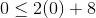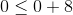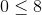This is true; select the side of this line that includes the origin.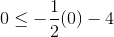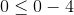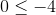This is false; select the side of this line that does not include the origin.

The solution sets of the individual inequalities are below: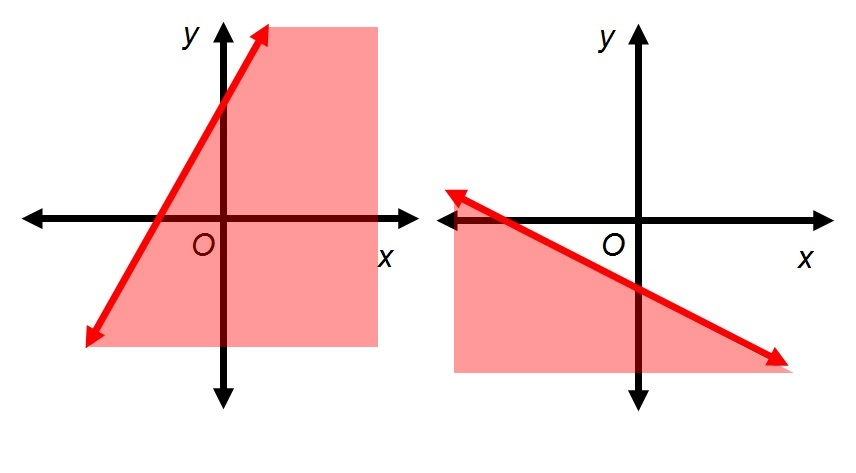The graph of the system is the intersection of the two sets, shown below:### Example Question #34 : How To Find The Equation Of A Line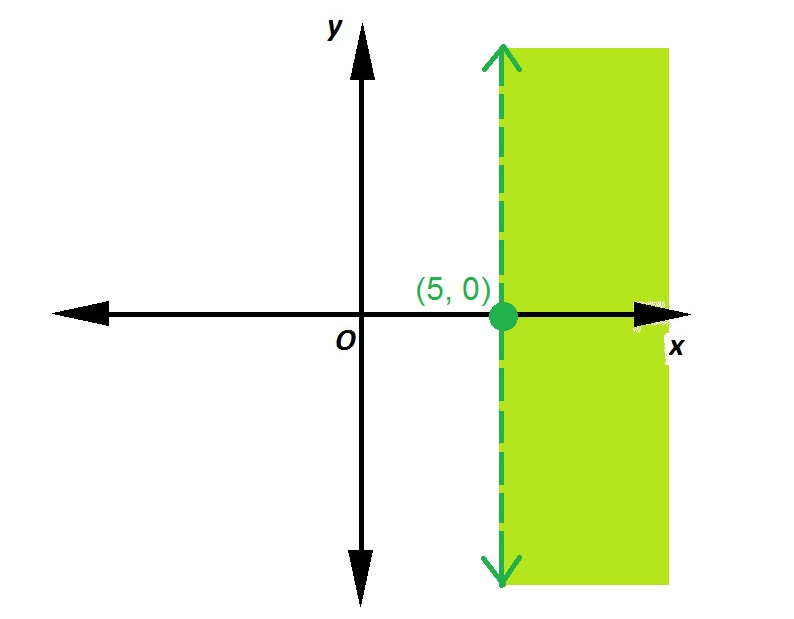Which of the following inequalities is graphed above?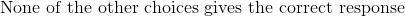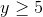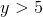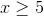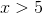Explanation:

The boundary line is a horizontal line which has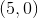as its-intercept; the equation of this line isThe inequality is eitheror, since the region right of the line is included. The dashed boundary indicates that equality is not allowed, so the correct inequality is.

### Example Question #35 : How To Find The Equation Of A Line

Find the equation of a line that passes through the following points: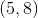and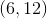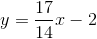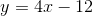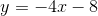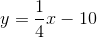Explanation:

Recall that the the following is the slope-intercept form of a line:In this equation, the variables are represented by the following: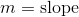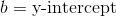Find the slope of the line by using the following formula: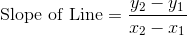In this equation, the x- and y-variables correspond to the coordinates of the given points.Next, find the y-intercept of the line by substituting one of the points into the semi-completed formula.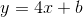Substituting in the pointyields the following: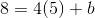Rearrange and solve for.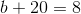Subtract 20 from both sides of the equation.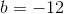Substitute this value of the y-intercept into our semi-complete equation to get the answer:1 2 4 Next →